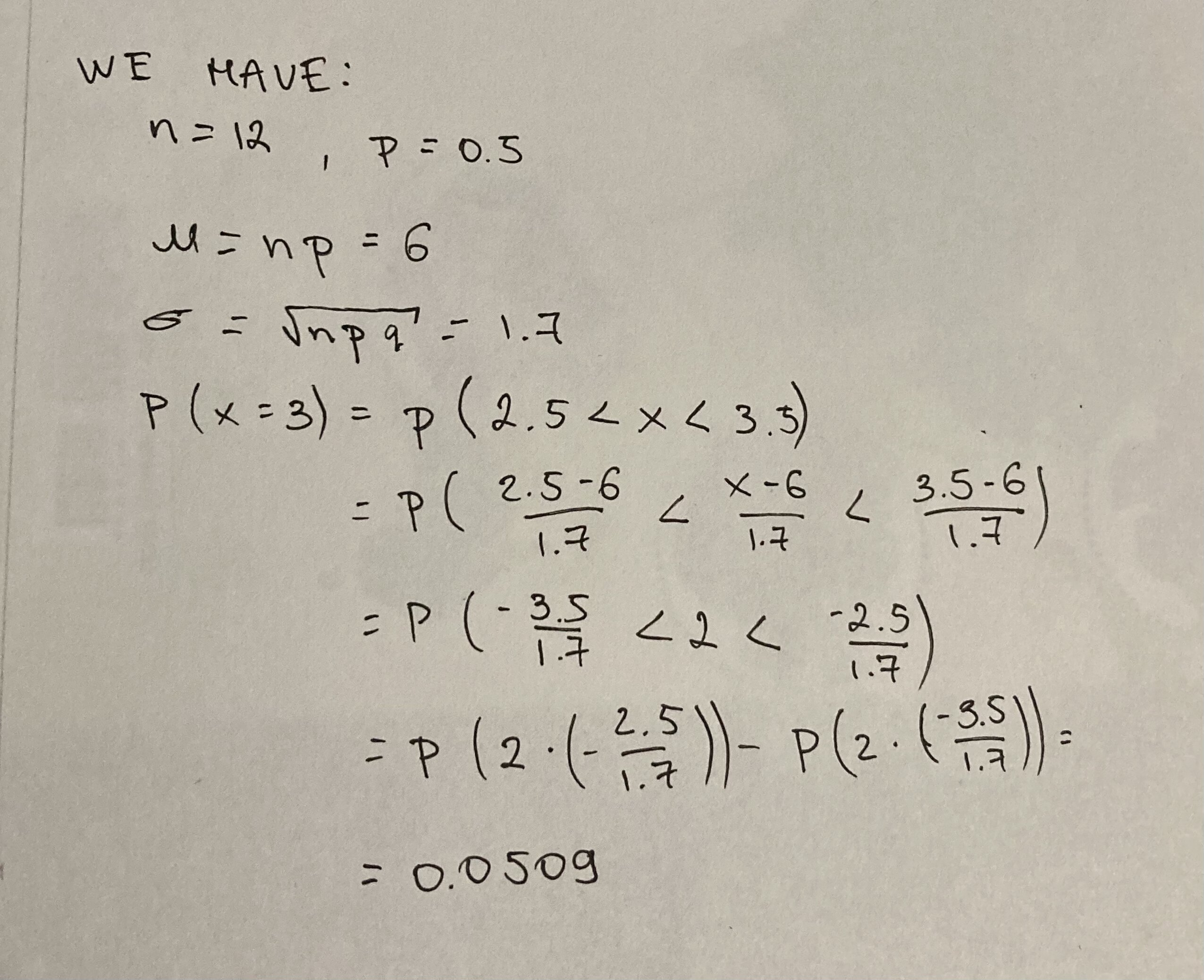Question# Please, find the normal approximation for the binomial probability of P(x = 3) where n = 12 and p = 0.5

Binomial probability
ANSWEREDPlease, find the normal approximation for the binomial probability of $$P(x = 3)$$ where $$n = 12$$ and $$p = 0.5$$